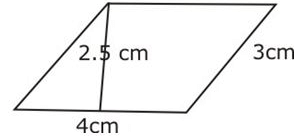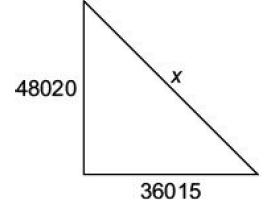Test: Mensuration- 4

# Test: Mensuration- 4

Test Description

## 15 Questions MCQ Test Quantitative Aptitude (Quant) | Test: Mensuration- 4

Test: Mensuration- 4 for Banking Exams 2023 is part of Quantitative Aptitude (Quant) preparation. The Test: Mensuration- 4 questions and answers have been prepared according to the Banking Exams exam syllabus.The Test: Mensuration- 4 MCQs are made for Banking Exams 2023 Exam. Find important definitions, questions, notes, meanings, examples, exercises, MCQs and online tests for Test: Mensuration- 4 below.
Solutions of Test: Mensuration- 4 questions in English are available as part of our Quantitative Aptitude (Quant) for Banking Exams & Test: Mensuration- 4 solutions in Hindi for Quantitative Aptitude (Quant) course. Download more important topics, notes, lectures and mock test series for Banking Exams Exam by signing up for free. Attempt Test: Mensuration- 4 | 15 questions in 15 minutes | Mock test for Banking Exams preparation | Free important questions MCQ to study Quantitative Aptitude (Quant) for Banking Exams Exam | Download free PDF with solutions
 1 Crore+ students have signed up on EduRev. Have you?
Test: Mensuration- 4 - Question 1

### Find the volume of a cuboid whose length is 8 cm, breadth 6 cm and height 3.5 cm.

Detailed Solution for Test: Mensuration- 4 - Question 1

Explanation:

Length of the cuboid = 8 cm

Breadth of the cuboid = 6 cm

Height of the cuboid = 3.5 cm

Volume of the cuboid = length × breadth × height

= 8 x 6 x 3.5 = 168cm3

Therefore,volume of  the cuboid = 168cm3

Test: Mensuration- 4 - Question 2

### Find the area of the given figure.Detailed Solution for Test: Mensuration- 4 - Question 2

Explanation:

Area of  a parallellogram =  Base x Height = 4 x 2.5 = 10 cm2

Test: Mensuration- 4 - Question 3

### Find the volume of a cuboid whose length is 8 cm, width is 3 cm and height is 5 cm.

Detailed Solution for Test: Mensuration- 4 - Question 3

:Length of  the cuboid = 8 cm

Width of the cuboid = 3 cm

Height of the cuboid = 5 cm

Volume of  a cuboid  = length × breadth × height

Therefore, Volume of the given cuboid = 8x3x5 = 120 cm3

Test: Mensuration- 4 - Question 4

Find the perimeter of a rectangle whose length is 4 cm and breadth is 3 cm.

Detailed Solution for Test: Mensuration- 4 - Question 4

Explanation:Length of the rectangle,l = 4cmBreadth of the rectangle,b = 3 cmPerimeter of a rectangle = 2(l+b) = 2(4+3) = 2x7 = 14 cm

Test: Mensuration- 4 - Question 5

Area of a rhombus = ______

Detailed Solution for Test: Mensuration- 4 - Question 5

Explanation:Area of a rhombus = Half the product of its diagonals                               = 1/2 x d1x d2

Test: Mensuration- 4 - Question 6

Area of a trapezium = Half of the sum of the lengths of parallel sides × ______

Detailed Solution for Test: Mensuration- 4 - Question 6

Explanation:Area of a trapezium =( Half of the sum of the length of parallel sides )×(perpendicular distance between them)

Test: Mensuration- 4 - Question 7

Surface area of a cuboid = __________

Detailed Solution for Test: Mensuration- 4 - Question 7

Explanation:Surface area of a cuboid = 2(lb + bh + hl)where, l= lengthb = breadthand h = height

Test: Mensuration- 4 - Question 8

_________ of a solid is the sum of the areas of its faces.

Detailed Solution for Test: Mensuration- 4 - Question 8

Explanation:The surface area of a three-dimensional figure is the sum of the areas of all its faces.

Test: Mensuration- 4 - Question 9

In a right angled triangle, find the hypotenuse if base and perpendicular are respectively 36015 cmand 48020 cm.

Detailed Solution for Test: Mensuration- 4 - Question 9Let hypotenuse = x cm
Then, by Pythagoras theorem:
x2 = (48020)2 + (36015)2
x fi 60025 cm

Test: Mensuration- 4 - Question 10

The inner circumference of a circular track is 440 cm. The track is 14 cm wide. Find the diameterof the outer circle of the track.

Detailed Solution for Test: Mensuration- 4 - Question 10

Let inner radius = A; then 2pr = 440 \ p = 70
Radius of outer circle = 70 + 14 = 84 cm
Outer diameter = 2 × Radius = 2 × 84 = 168

Test: Mensuration- 4 - Question 11

A pit 7.5 metre long, 6 metre wide and 1.5 metre deep is dug in a field. Find the volume of soilremoved in cubic metres.

Detailed Solution for Test: Mensuration- 4 - Question 11

Volume of soil removed = l × b × h
= 7.5 × 6 × 1.5 = 67.5 m3

Test: Mensuration- 4 - Question 12

The whole surface of a rectangular block is 8788 square cm. If length, breadth and height are inthe ratio of 4 : 3 : 2, find length.

Detailed Solution for Test: Mensuration- 4 - Question 12

Let the common ratio be = x
Then, length = 4x, breadth = 3x and height = 2x
As per question;
2(4x x 3x + 3x x 2x + 2x x 4x) = 8788
2(12x2 + 6x2 + 8x2) = 8788 fi 52x2 = 8788
fi x = 13
Length = 4x = 52 cm

Test: Mensuration- 4 - Question 13

Find the height of a Cuboid , if the volume and area of its base is 1240cm3 and 40 sq.cm respectively.

Test: Mensuration- 4 - Question 14

A bicycle wheel makes 5000 revolutions in moving 11 km. What is the radius of the wheel?

Detailed Solution for Test: Mensuration- 4 - Question 14

Let the radius of the wheel be = p
Then 5000 × 2pr = 1100000 cm fi r = 35 cm

Test: Mensuration- 4 - Question 15

The short and the long hands of a clock are 4 cm and 6 cm long respectively. What will be sum ofdistances travelled by their tips in 4 days? (Take p = 3.14)

Detailed Solution for Test: Mensuration- 4 - Question 15

In 4 days, the short hand covers its circumference
4 × 2 = 8 times long hand covers its circumference
4 × 24 = 96 times
Then they will cover a total distance of:-
(2 × p × 4)8 + (2 × p × 6)96 fi 3818.24 cm

## Quantitative Aptitude (Quant)

163 videos|163 docs|131 tests
 Use Code STAYHOME200 and get INR 200 additional OFF Use Coupon Code
Information about Test: Mensuration- 4 Page
In this test you can find the Exam questions for Test: Mensuration- 4 solved & explained in the simplest way possible. Besides giving Questions and answers for Test: Mensuration- 4, EduRev gives you an ample number of Online tests for practice

## Quantitative Aptitude (Quant)

163 videos|163 docs|131 tests